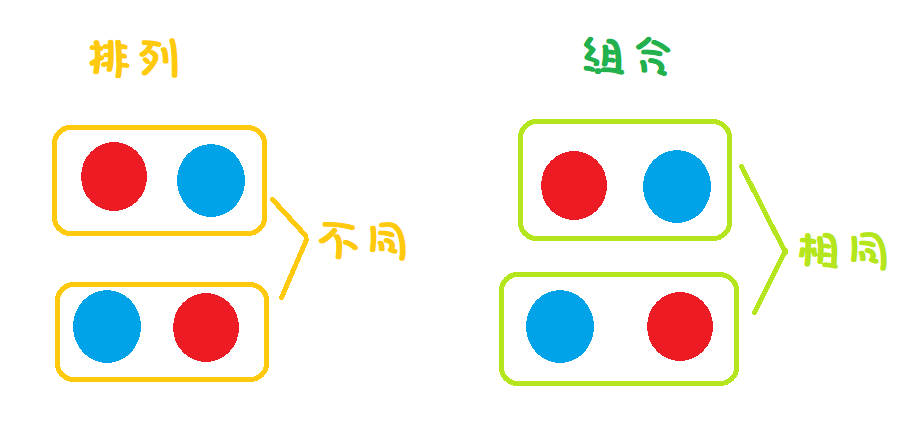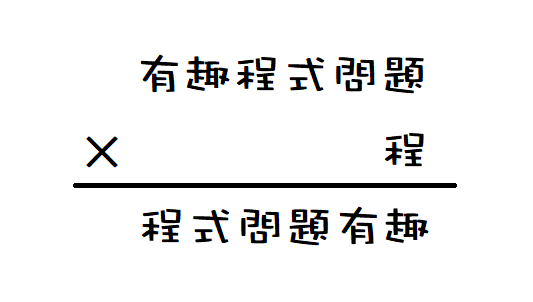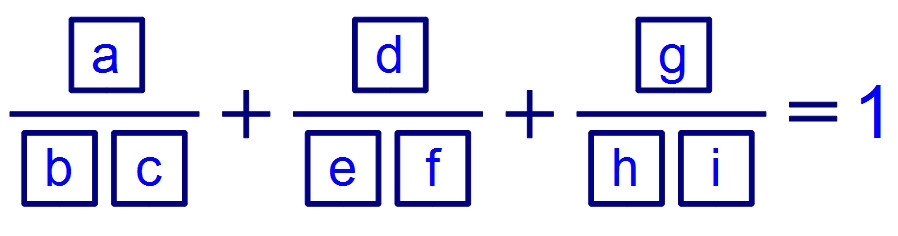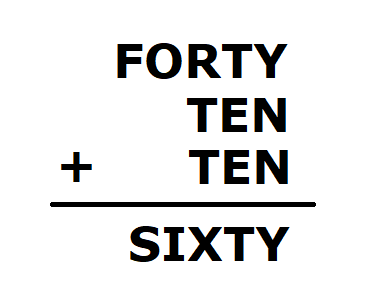DAY 26
3
Software Development

Day26- python內建itertools模組簡介，窮舉排列組合

(還沒看過題目的朋友歡迎點昨日題目傳送門)

昨日課後練習討論

def trans(article):
return ''.join([c.lower() if c.isalpha() else ' ' for c in article])

方便窮舉排列組合的itertools

itertools可以非常方便的幫我們解決排列組合上的問題:

排列、組合傻傻分不清?

「排列」指的是取出元素的順序不同就視為是不同的狀況，

「先取紅球再取藍球」與「先取藍球再取紅球」是不同的情況，itertools 的使用

排列

from itertools import permutations
print(list(permutations("ABCD",2)))

組合

from itertools import combinations
print(list(combinations("ABCD",2)))

元素可重複的組合

from itertools import combinations_with_replacement
print(list(combinations_with_replacement("ABCD",2)))

笛卡兒積

from itertools import product
print(list(product('ABC', 'xyz')))

(其中本文特別講「排列」的運用)

範例26-1 有趣程式問題def tupleToNum(Tuple):
return int(''.join(map(str,Tuple)))
def check(Tuple):
return tupleToNum(Tuple)*Tuple==tupleToNum(Tuple[2:]+Tuple[:2])
for p in permutations(range(1,10),6):
if check(p):
print(p)

tupleToNum函數是將一個元組轉換成數字的形式，

check函數是檢查將數字代入有趣程式問題是否滿足等式。
for迴圈則是窮舉所有排列，若找到解則印出來。

範例26-2 三分數之和等於1from itertools import permutations

def tupleToFloat(Tuple):
return Tuple/(Tuple*10+Tuple)
def check(Tuple):
return tupleToFloat(Tuple[0:3])+tupleToFloat(Tuple[3:6])+tupleToFloat(Tuple[6:9])==1
for p in permutations(range(1,10),9):
if check(p):
print(p)

tupleToNum函數是將一個元組轉換成浮點數的形式，

check函數用來檢查每組答案是否滿足等式。

(5, 3, 4, 7, 6, 8, 9, 1, 2)
(5, 3, 4, 9, 1, 2, 7, 6, 8)
(7, 6, 8, 5, 3, 4, 9, 1, 2)
(7, 6, 8, 9, 1, 2, 5, 3, 4)
(9, 1, 2, 5, 3, 4, 7, 6, 8)
(9, 1, 2, 7, 6, 8, 5, 3, 4)

課後練習1 則留言

1

from itertools import permutations
print(list(permutations("ABCD",2)))

from itertools import combinations
print(list(combinations("ABCD",2)))